# Measuring Density with Laboratory Balance

## Use Your Balance and a Density Kit to Determine the Density of Solid, Liquid and Viscous SubstancesThe solid is weighed in air (A) and then again (B) in the auxiliary liquid with a known density. The density of the solid ρ can be calculated as follows: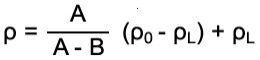ρ        = Density of the sample

A        = Weight of the sample in air

B        = Weight of the sample in the auxiliary liquid

ρ0       = Density of the auxiliary liquid

ρL       = Density of air

The temperature of the liquid must be taken into account as this can cause density changes of the order of magnitude 0.001 to 0.1 per °C, the effect
of which can be seen in the third decimal place of the result.

Gravimetric, BuoyancyGravimetric, DisplacementPycnometerDigital Density Meter
MethodsBeaker for auxiliary liquid stands on a platform or below the balance.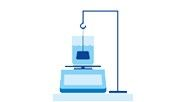Beaker for auxiliary liquid stands on the balance.Glass beaker of defined volume.Oscillating tube technology
Suitable for
• Solids
• Liquids (with glass sinker)
• Pasty substances(with gamma sphere)
• Liquids (with glass sinker)
Solids
• Liquids, Dispersions
• Powder
• Granules
• Liquids
• Gases
• Quick process
• Flexible with regards to sample size
• Quick process
• Accurate method
• Quick process
• Exact temperature control by Peltier elements
• Automatic density measurement
• Small sample volumes
• Temperature sensitive
• Sample must be wet very carefully
• Air bubbles must not be trapped
• Temperature sensitive
• Large sample volume required
• Temperature sensitive
• Labour-intensive
• Time-consuming
• Air bubbles must not be trapped
• Viscous samples require a viscosity correction (available in modern instruments).

If you know the mass and volume of a sample (solids or liquids), its density can be calculated from: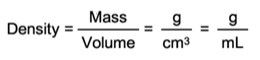The Difficulty with Volume

It's quite simple to weigh a sample accurately, but determining the volume of a sample accurately can be challenging.

Buoyancy

The buoyancy method avoids the problem of determining volume because it involves weighing the sample twice in two different mediums (air and a liquid). The volume can therefore be assumed to be constant in both situations.

Displacement

In the simplest application of the displacement method, the volume of a solid sample is determined by observing the increase in the level of the liquid in which the sample is submerged.
Conversely, when an object of known volume is submerged in a liquid with unknown density, the difference in the weight values (in air and in the liquid) can be used to determine the density of the liquid.

Pycnometer

A pycnometer is a specially designed glass flask, usually with a defined volume. It is most often used to determine the density of liquids. The pycnometer is first weighed empty and then full of the liquid under investigation. The difference (i.e. the mass of the sample) divided by the volume of the pycnometer is the density of the sample.
The pycnometer method can also be used for determining the density of powder samples or granulates.

Digital Density Meter

A hollow glass tube vibrates at a certain frequency. The frequency changes when the tube is filled with different substances: the higher the mass of the sample, the lower the frequency. Digital density meters work by measuring the frequency and converting it into density.

Please see the table below for a comparison of these four different methods.

Gravimetric, BuoyancyGravimetric, DisplacementPycnometerDigital Density Meter
MethodsBeaker for auxiliary liquid stands on a platform or below the balance.Beaker for auxiliary liquid stands on the balance.Glass beaker of defined volume.Oscillating tube technology
Suitable for
• Solids
• Liquids (with glass sinker)
• Pasty substances(with gamma sphere)
• Liquids (with glass sinker)
Solids
• Liquids, Dispersions
• Powder
• Granules
• Liquids
Measuring principle for
a solid sample
The sample is weighed once in air and once immersed in the auxiliary liquid with known density.

Density of the solid sample can be determined from the known density of the liquid and the two mass values.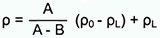ρ,= Density of the sample
A,= Weight of the sample in air
B,= Weight of the sample in the auxiliary liquid
ρ0,= Density of the auxiliary liquid
ρL,= Density of air
The auxiliary liquid with known density is weighed before and after immersing the sample (tare can be used to measure mass difference directly).

Using the mass difference and the liquid density the volume of the sample can be determined. This is then used together with the mass of the sample to calculate its density.
The pycnometer is first weighed empty and then again full with the reference liquid of known density. The powder is added to the cleaned and dried pycnometer. It is weighed to determine the weight of the powder sample. The pycnometer is then topped up with the same liquid in which the powder must be completely insoluble. The pycnometer is weighed again. The weight of the displaced liquid can then be determined, and hence the density of the powder calculated.n/a
Measuring principle for
a liquid sample
The reference body with known volume (glass sinker) is weighed once in air and once in the liquid with unknown density.

Density of the liquid can be determined from the known volume of the reference body and the two mass values.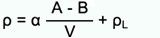ρ,= Density of the liquid sample
α,= Weight correction factor (0.99985), to take the atmospheric buoyancy of the adjustment weight into account
A,= Weight of the reference body in air
B,= Weight of the reference body in the liquid
V,= Known volume of the reference body
ρL,= Density of air
The weight of the liquid with the unknown density is measured before (tare) and after immersing the reference body (gamma sphere or glass sinker).

Using the mass difference and the known volume of the reference body, the density of the liquid sample can be determined.
The pycnometer is first weighed empty and then again full with the liquid sample. The mass difference divided by the volume of the pycnometer is the density of the liquid.The sample is added to a U-shaped hollow glass tube in the device. The density of the sample is determined by measuring the frequency of vibration of the tube. The lower the frequency of vibration, the higher the density of the sample.Density Standards

Many standards and norms exist for the determination of density in solids samples. Some of the most commonly used are:

• ISO 1183-1: Plastics — Methods for determining the density of non-cellular plastics
• OIML G 14: Density measurement according OIML
• ASTM-D-792: Standard Test Methods for Density and Specific Gravity Standard

ISO 1183-1 specifies the use of a 4-decimal place analytical balance.

The Confusion with Bulk Density

Bulk density is a measure of how many particles, parts or pieces are contained within a measured volume. Bulk density is not a property of the material itself. Bulk density includes the spaces between the particles or items as well as any voids within the items themselves. Bulk density can vary depending on how the material is handled; shaking a container, for example, allows parts to settle, increasing the overall bulk density.### Moisture Content Determination# Extended Data Management and Process Security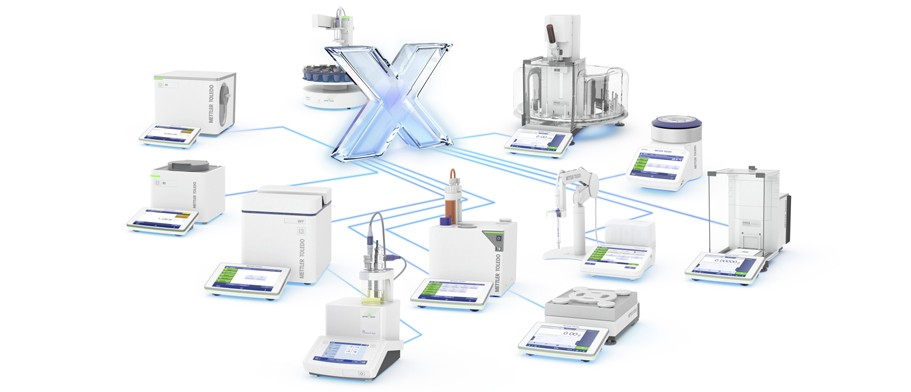The combination of a METTLER TOLEDO Excellence level balance and LabX software offers a higher level of data management and process security. Excellence analytical and precision balances can be set up with a Density Kit for density determination. LabX ensures that your density SOP is followed precisely. LabX records all weight values, performs all calculations and saves all results securely in a central database. All the data related to your density application can be transferred directly to your internal data management system.